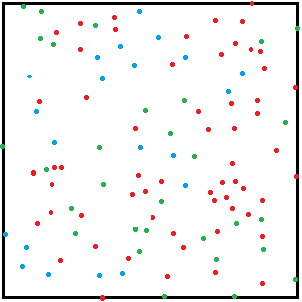# Squares just got a whole lot serious

Calculus Level 3If the average distance from a point randomly selected in the unit square to its center equals $\frac{\ln(a+\sqrt{b})+\sqrt{b}}{c}$ where $a,b$ and $c$ are positive integers with $b$ being square-free. Find $a+b+c$.

×

Problem Loading...

Note Loading...

Set Loading...